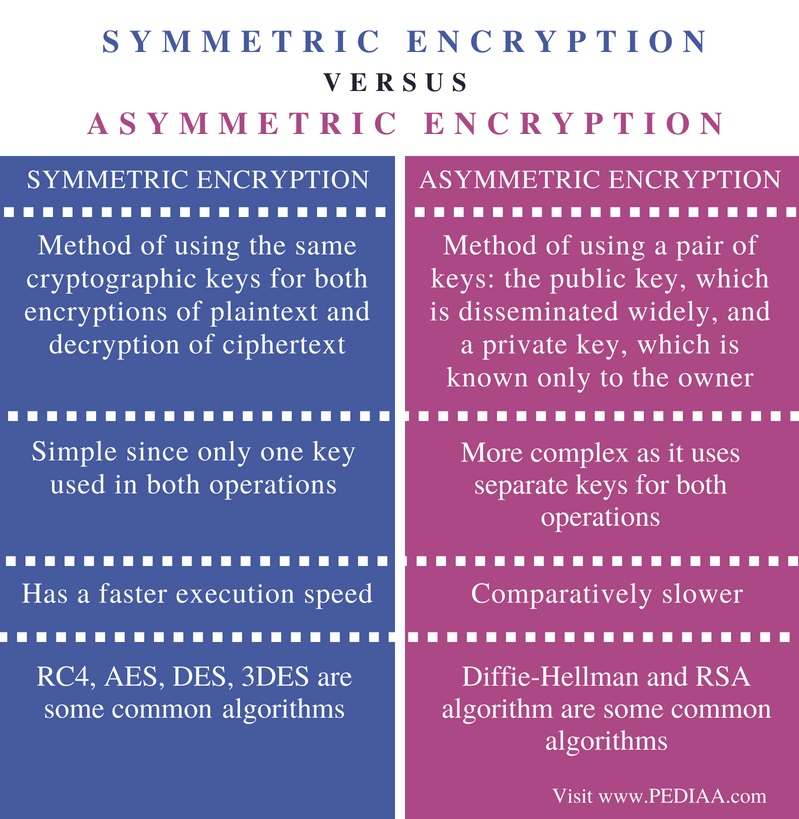# Difference Between Symmetric and Asymmetric Encryption

The main difference between symmetric and asymmetric encryption is that the symmetric encryption uses the same key for both encryption and decryption while the asymmetric encryption uses two different keys for encryption and decryption.

It is important to ensure that information shared over the internet is secure. It becomes more vital when exchanging confidential data. Cryptography is a technique that helps to pass information securely in communication. It consists of two major functionalities called encryption and decryption. Encryption occurs at the sender’s end. In encryption, the original message is transformed into an unrecognizable message so that hackers cannot identify the content of the message. Decryption occurs at the receiver’s end. In decryption, the received message is transformed back to the original message. This way, encryption and decryption help to secure data in communication. Symmetric and asymmetric encryption are two techniques used in encryption and decryption.

### Key Areas Covered

1. What is Symmetric Encryption
– Definition, Functionality
2. What is Asymmetric Encryption
– Definition, Functionality
3. Difference Between Symmetric and Asymmetric Encryption
– Comparison of Key Differences

### Key Terms

Asymmetric Encryption, Cryptography, Decryption, Encryption, Symmetric Encryption## What is Symmetric Encryption

In symmetric encryption, the same key is used for both encryption and decryption. In this method, the original message is converted into an unrecognizable message which cannot be identified. This converted message is called the ciphertext. This is done using a key and an encrypting algorithm. At the receiving end, the ciphertext is transformed back to the original message using the same key and a decryption algorithm.

As both parties use the same key, symmetric encryption is much faster. On the other hand, the key must be available to decrypt the message. Therefore, a secure channel is required to transfer the key. Overall, symmetric encryption is a straightforward method and does not require much time to complete. RC4, AES, DES, 3DES are some common symmetric encryption algorithms.

## What is Asymmetric Encryption

Asymmetric encryption uses two keys for encryption and decryption. These two keys are called the private key and public key.  It uses the public key for encryption and the private key for decryption. The public key is available for a party who wish to send a message. The private key belongs to the owner of the message. A message that is encrypted by a public key and an encryption algorithm can be decrypted using the decryption algorithm and the matching private key of the corresponding public key.Overall, asymmetric encryption provides more data security. On the other hand, the involvement of two keys makes asymmetric encryption time consuming and more complex. Diffie-Hellman and RSA algorithm are some common Asymmetric Encryption algorithms.

## Difference Between Symmetric and Asymmetric Encryption

### Definition

Symmetric encryption is the method of using the same cryptographic keys for both encryptions of plaintext and decryption of ciphertext. Asymmetric encryption is the method of using a pair of keys: the public key, which is disseminated widely, and a private key, which is known only to the owner.

### Complexity

Since only one key used in both operations, symmetric encryption is simple.  However, asymmetric encryption is more complex as it uses separate keys for both operations.

### Execution Speed

Asymmetric encryption is slower compared to symmetric encryption, which has a faster execution speed.

### Algorithms

RC4, AES, DES, 3DES are some common Symmetric Encryption algorithms. Diffie-Hellman and RSA algorithm are some common Asymmetric Encryption algorithms.

### Conclusion

Symmetric and asymmetric encryption are two techniques used in encryption and decryption. The difference between symmetric and asymmetric encryption is that symmetric encryption uses the same key for both encryption and decryption while asymmetric encryption uses two different keys for encryption and decryption.

##### Reference:

1.. Symmetric Key and Public Key Encryption, Itfreetraining, 18 June 2013, Available here.

##### Image Courtesy:

1. “Cryptography Encryption Privacy” (CC0) via Maxpixel.com